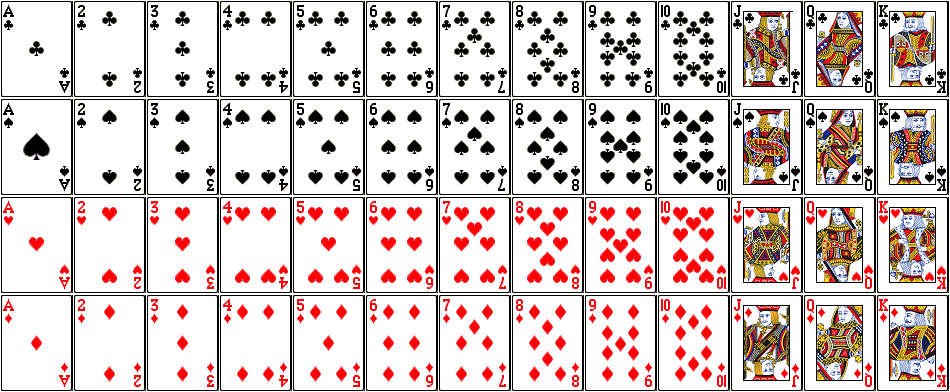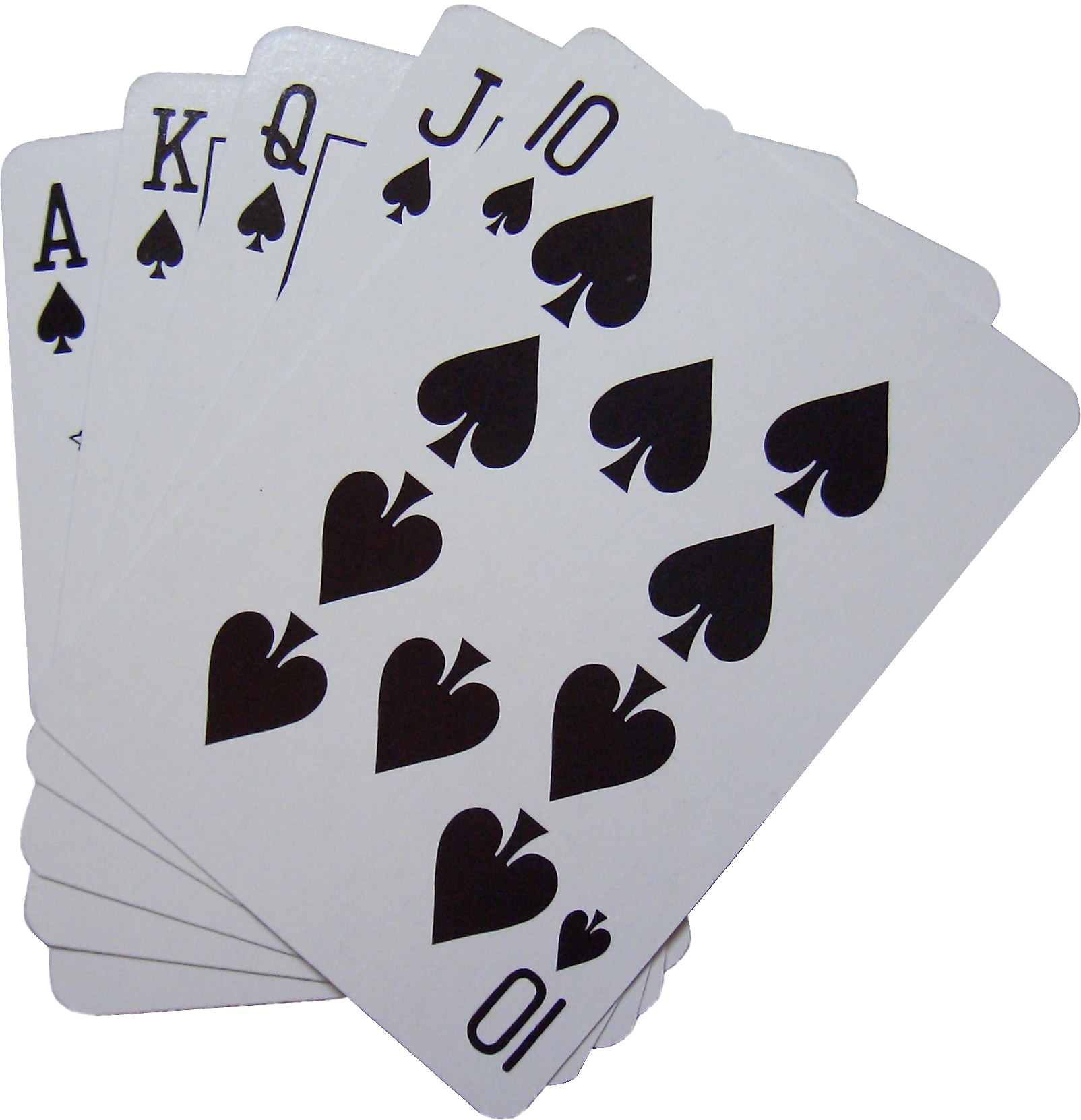Game Theory (Part 10)

Game Theory (Part 10)

John Baez

Last time we solved some probability puzzles involving coin flips. This time we'll look at puzzles involving cards.

Permutations

Example 1. How many ways are there to order 3 cards: a jack (J), a queen (Q), and a king (K)?

By order them I mean put one on top, then one in the middle, then one on the bottom. There are three choices for the first card: it can be A, Q, or K. That leaves two choices for what the second card can be, and just one for the third. So, there are

$$3 \times 2 \times 1 = 6$$

ways to order the cards.

Example 2. How many ways are there to order all 52 cards in an ordinary deck?

By the same reasoning, the answer is

$$52 \times 51 \times 50 \times \cdots \times 2 \times 1$$

This is a huge number. We call it 52 factorial, or 52! for short. I guess the exclamation mark emphasizes how huge this number is. In fact

$$52! \approx 8.06 \times 10^{67}$$

This is smaller than the number of atoms in the observable universe, which is about $$10^{80}.$$ But it's much bigger than the number of galaxies in the observable universe, which is about $$10^{11}$$, or even the number of stars in the observable universe, which is roughly $$10^{22}.$$ It's impressive that we can hold such a big number in our hand... in the form of possible ways to order a deck of cards!

A well-shuffled deck

Definition 1. We say a deck is well-shuffled if each of the possible ways of ordering the cards in the deck has the same probability.

Example 3. If a deck of cards is well-shuffled, what's the probability that it's in this order?Since all orders have the same probability, and there are $$52!$$ of them, the probability that they're in any particular order is

$$\displaystyle{ \frac{1}{52!} }$$

$$\displaystyle{ \frac{1}{52!} \approx 1.24 \times 10^{-68} }$$

A hand from a well-shuffled deck

Suppose you take the top $$k$$ cards from a well-shuffled deck of $$n$$ cards. You'll get a subset of cards—though card players call this a hand of cards instead of a subset. And, there are $$n$$ choose $$k$$ possible hands you could get! Remember from last time:

Definition 2. The binomial coefficient

$$\displaystyle{ \binom{n}{k} = \frac{n(n-1)(n-2) \cdots (n-k+1)}{k(k-1)(k-2) \cdots 1}}$$

called $$n$$ choose $$k$$ is the number of ways of choosing a subset of $$k$$ things from a set of $$n$$ things.

I guess card-players call a set a 'deck', and a subset a 'hand'. But now we can write a cool new formula for $$n$$ choose $$k.$$ Just multiply the top and bottom of that big fraction by

$$\displaystyle{ (n-k)(n-k-1) \cdots 1}$$

We get

$$\begin{array}{ccl} \displaystyle{ \binom{n}{k}} &=& \displaystyle{ \frac{n(n-1)(n-2) \cdots 1}{(k(k-1)(k-2) \cdots 1)((n-k)(n-k-1) \cdots 1)} } \\ &=& \displaystyle{ \frac{n!}{k! (n-k)!} } \end{array}$$

I won't do it here, but here's something you can prove using stuff I've told you. Suppose you have a well-shuffled deck of $$n$$ cards and you draw a hand of $$k$$ cards. Then each of these hands is equally probable!

Using this we can solve lots of puzzles.

Example 4. If you draw a hand of 5 cards from a well-shuffled standard deck, what's the probability that you get the 10, jack, queen, king and ace of spades?

Since I'm claiming that all hands are equally probable, we just need to count the number of hands, and take the reciprocal of that.

There are

$$\displaystyle{ \binom{52}{5} = \frac{52 \times 51 \times 50 \times 49 \times 48}{5 \times 4 \times 3 \times 2 \times 1} }$$

5-card hands drawn from a 52-card deck. So, the probability of getting any particular hand is

$$\displaystyle{ \frac{1}{\binom{52}{5}} = \frac{5 \times 4 \times 3 \times 2 \times 1}{52 \times 51 \times 50 \times 49 \times 48} }$$

We can simplify this a bit since 50 is 5 × 10 and 48 is twice 4 × 3 × 2 × 1. So, the probability is

$$\displaystyle{ \frac{1}{52 \times 51 \times 10 \times 49 \times 2} = \frac{1}{2598960} \approx 3.85 \cdot 10^{-7}}$$

A royal flushThe hand we just saw:

{10♠, J♠, Q♠, K♠, A♠}

is an example of a 'royal flush'... the best kind of hand in poker!

Definition 3. A straight is a hand of five cards that can be arranged in a consecutive sequence, for example:

{7♥, 8♣, 9♠, 10♠, J♦}

Definition 4. A straight flush is a straight whose cards are all of the same suit, for example:

{7♣, 8♣, 9♣, 10♣, J♣}

Definition 5. A royal flush is a straight flush where the cards go from 10 to ace, for example:

{10♠, J♠, Q♠, K♠, A♠}

Example 5. If you draw a 5-card hand from a standard deck, what is the probability that it is a royal flush?

We have seen that each 5-card hand has probability

$$\displaystyle{ \frac{1}{\binom{52}{5}} = \frac{1}{2598960} }$$

There are just 4 royal flushes, one for each suit. So, the probability of getting a royal flush is

$$\displaystyle{ \frac{4}{\binom{52}{5}} = \frac{1}{649740} \approx 0.000154\%}$$

Puzzles

Suppose you have a well-shuffled standard deck of 52 cards, and you draw a hand of 5 cards.

Puzzle 1. What is the probability that the hand is a straight flush?

Puzzle 2. What is the probability that the hand is a straight flush but not a royal flush?

Puzzle 3. What is the probability that the hand is a straight?

Puzzle 4. What is the probability that the hand is a straight but not a straight flush?